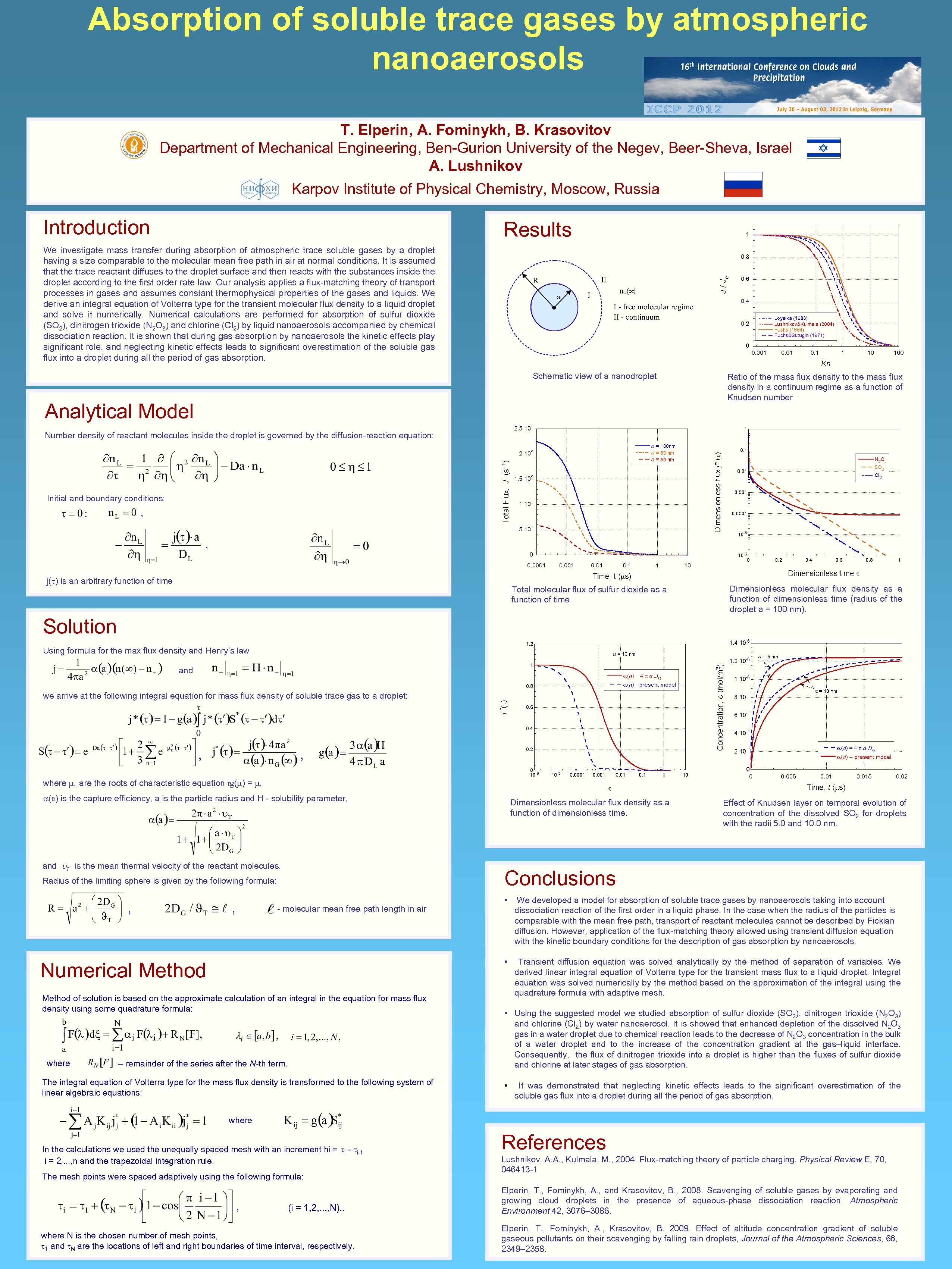Скачать презентацию Absorption of soluble trace gases by atmospheric nanoaerosols

68d3f388c8f3b2a26cabda7e1607778a.ppt

• Количество слайдов: 1Absorption of soluble trace gases by atmospheric nanoaerosols T. Elperin, A. Fominykh, B. Krasovitov Department of Mechanical Engineering, Ben-Gurion University of the Negev, Beer-Sheva, Israel A. Lushnikov Karpov Institute of Physical Chemistry, Moscow, Russia Introduction Results We investigate mass transfer during absorption of atmospheric trace soluble gases by a droplet having a size comparable to the molecular mean free path in air at normal conditions. It is assumed that the trace reactant diffuses to the droplet surface and then reacts with the substances inside the droplet according to the first order rate law. Our analysis applies a flux-matching theory of transport processes in gases and assumes constant thermophysical properties of the gases and liquids. We derive an integral equation of Volterra type for the transient molecular flux density to a liquid droplet and solve it numerically. Numerical calculations are performed for absorption of sulfur dioxide (SO 2), dinitrogen trioxide (N 2 O 3) and chlorine (Cl 2) by liquid nanoaerosols accompanied by chemical dissociation reaction. It is shown that during gas absorption by nanoaerosols the kinetic effects play significant role, and neglecting kinetic effects leads to significant overestimation of the soluble gas flux into a droplet during all the period of gas absorption. Schematic view of a nanodroplet Analytical Model Ratio of the mass flux density to the mass flux density in a continuum regime as a function of Knudsen number Number density of reactant molecules inside the droplet is governed by the diffusion-reaction equation: Initial and boundary conditions: , , j( ) is an arbitrary function of time Total molecular flux of sulfur dioxide as a function of time Dimensionless molecular flux density as a function of dimensionless time (radius of the droplet a = 100 nm). Dimensionless molecular flux density as a function of dimensionless time. Effect of Knudsen layer on temporal evolution of concentration of the dissolved SO 2 for droplets with the radii 5. 0 and 10. 0 nm. Solution Using formula for the max flux density and Henry’s law and we arrive at the following integral equation for mass flux density of soluble trace gas to a droplet: , , where n are the roots of characteristic equation tg( ) = , (a) is the capture efficiency, a is the particle radius and H - solubility parameter, and T is the mean thermal velocity of the reactant molecules. Conclusions Radius of the limiting sphere is given by the following formula: , - molecular mean free path length in air Numerical Method of solution is based on the approximate calculation of an integral in the equation for mass flux density using some quadrature formula: where – remainder of the series after the N-th term. The integral equation of Volterra type for the mass flux density is transformed to the following system of linear algebraic equations: We developed a model for absorption of soluble trace gases by nanoaerosols taking into account dissociation reaction of the first order in a liquid phase. In the case when the radius of the particles is comparable with the mean free path, transport of reactant molecules cannot be described by Fickian diffusion. However, application of the flux-matching theory allowed using transient diffusion equation with the kinetic boundary conditions for the description of gas absorption by nanoaerosols. • , • Transient diffusion equation was solved analytically by the method of separation of variables. We derived linear integral equation of Volterra type for the transient mass flux to a liquid droplet. Integral equation was solved numerically by the method based on the approximation of the integral using the quadrature formula with adaptive mesh. • Using the suggested model we studied absorption of sulfur dioxide (SO 2), dinitrogen trioxide (N 2 O 3) and chlorine (Cl 2) by water nanoaerosol. It is showed that enhanced depletion of the dissolved N 2 O 3 gas in a water droplet due to chemical reaction leads to the decrease of N 2 O 3 concentration in the bulk of a water droplet and to the increase of the concentration gradient at the gas–liquid interface. Consequently, the flux of dinitrogen trioxide into a droplet is higher than the fluxes of sulfur dioxide and chlorine at later stages of gas absorption. • It was demonstrated that neglecting kinetic effects leads to the significant overestimation of the soluble gas flux into a droplet during all the period of gas absorption. where In the calculations we used the unequally spaced mesh with an increment hi = i - i-1 i = 2, . . . , n and the trapezoidal integration rule. The mesh points were spaced adaptively using the following formula: , (i = 1, 2, . . . , N). . where N is the chosen number of mesh points, 1 and N are the locations of left and right boundaries of time interval, respectively. References Lushnikov, A. A. , Kulmala, M. , 2004. Flux-matching theory of particle charging. Physical Review E, 70, 046413 -1 Elperin, T. , Fominykh, A. , and Krasovitov, B. , 2008. Scavenging of soluble gases by evaporating and growing cloud droplets in the presence of aqueous-phase dissociation reaction. Atmospheric Environment 42, 3076– 3086. Elperin, T. , Fominykh, A. , Krasovitov, B. 2009. Effect of altitude concentration gradient of soluble gaseous pollutants on their scavenging by falling rain droplets, Journal of the Atmospheric Sciences, 66, 2349– 2358.